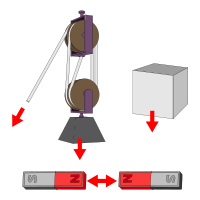# Force Calculator

To find magnitude of net force by putting values in input box by using our net force calculator.

Formula Force = Mass * Acceleration

kg

m/s²

N## Formula

Force :
F = m*a
m = F/a

Mass :
Acceleration :

a = F/m

Where,
m = Mass,
a = AccelerationCalculate the magnitude of force using our net force calculator. Force is defined as an influence that causes an object to change speed, direction, or shape. it's a physics term and this calculator is also known as the newton calculator.

## What is the force?

In physics, a force refers to the pushing or pulling of an object with mass to acceleration. In science, force is described as a quantitative description of the interaction between two physical bodies.Force is the derivative of momentum to time. Gravitation and electromagnetic force are those forces that can travel even across the empty vacuum of space.

Force is denoted by the symbol 'F'. Its SI unit is Newton represented symbolically with a capital “n” i.e N.

### Daily life examples:

We are using force every passing second. Earth has held us to the ground by gravitational force. We walk on earth using the force of friction. The building is standing using normal force.

Ball going back after colliding with bat due to impact force. Pressing a thumb pin on notice board using the pushing effect of force and many more

### Formula:

You can calculate the magnitude of force by using this formula or even by the calculator. The normal force of an object can also be calculated by this equation. It is called the force equation.

Force : F = ma

Where,

m = Mass,

a = Acceleration

Other units used for force are dyne, pound, or kilogram-force.

## Types of force

There are so many types of forces. Some of them are normal force, centripetal force, air resistance (friction), gravitational force, impact force, and applied force. Calculators are available online for easy calculation of these forces.

## How to find force manually?

Force can be calculated manually quite easily. The following are the steps you will need to follow.

1. Calculation of mass if not provided.

For this, divide the newtons by acceleration rate to get the value of mass.                                                                      m=Fa

2. Calculation of acceleration if not given. Use the formula written below.                                                                                a=Fm
3. Conversion of values of mass and acceleration into SI units. I.e kg and ms-2respectively.
4. Put the values in the force formula and calculate.

## Use our Force calculator

If you face any problem while calculating force OR you don't understand how to calculate the magnitude of force manually, Use our online magnitude of force calculator.

This advanced online Force Calculator of physics is used to calculate and find the force when the mass and acceleration of the object are known. Individual forces can be then added to find the Net force.

## Net force

When more than one bodies act on an object then the total force that causes motion or any change in the body is called Net force.

An example of the net force can be this: Consider two forces F1 and F2 of 10N and 20N respectively act from opposite sides on a body of mass 5kg. The acceleration produced will be 10 ms-2.

## How to find net force?

The net force consists of both magnitude and direction that makes it a vector quantity. So it can be calculated by the vector addition method.

As per Newton's second law, the net force acting upon an object is equal to the rate at which its momentum changes. The net force is also known as the resultant force.

Net or resultant force calculators are also available that provide results with direction.

### Example:

Calculate force for the given details of an object.

Mass (m) = 15 kg

Acceleration (a) = 10 ms-2

Solution:

Apply Formula:

F = m*a

F = 15*10

F = 150 N

Force (F) = 150 N

1. What is the force? Education.vic.gov.au.
2. What Is a Force in Science?ThoughtCo.